# Stationary/Standing Wave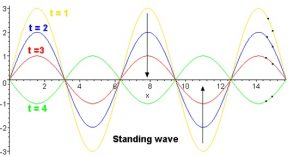Stationary or Standing wave
When two progressive wave of the same frequency and amplitude, travelling through a medium with the same speed but in opposite direction superimpose on each other and they give rise to a wave called stationary wave. In stationary wave, it does not seem to be moving and there is no net flow of energy, along the wave.

When a stationary wave is formed due to the super position of the two waves of equal frequency and amplitude travelling in opposite direction, the points of maximum and zero amplitude are resulted alternatively. The points where amplitude is maximum are called anti nodes (AN) and those with zero amplitude are called nodes (N).

Consider a progressive wave travelling in positive X-axis and another wave travelling in X-axis the equation of the wave travelling along positive X-axis is given by,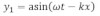The equation of the wave travelling along negative X-axis is given by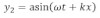According to the super position principle, the displacement of resultant wave is given by,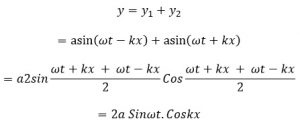This is the displacement of resultant wave and A= 2aCoskx is the amplitude of resultant wave.

This implies that the amplitude of the stationary wave different at different points i.e. amplitude become zero at some points and maximum at some other points.

Condition for maximum amplitude:

We wave,
The amplitude of resultant wave,
A= 2acoskx
For amplitude to be maximum,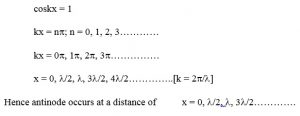Condition for minimum amplitude: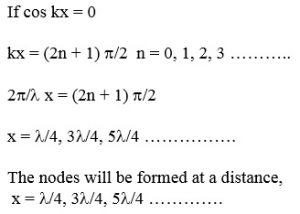Characteristics of stationary wave:

1. The stationary wave are produced when two progressive waves of equal frequency and amplitude travel in medium in opposite direction.
2. In the stationary wave, the disturbance or energy is not transmitted from particle to particle.
3. At nods, the particles of the medium are permanently at rest.
4. The particle at the anti-nodes vibrates with the maximum amplitude which is equal to twice the amplitude of either waves.
5. The period of vibration of stationary wave is equal to that of either of wave.
6. The amplitude of particles on either side of an antinode gradually decreases to zero.